Next: General comments Up: Examples Previous: Example 3   Contents

Example 4

In this example (see section 15.1.3) we deal with a complex problem of three equations in three unknowns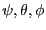. We are looking for a solution in the domain: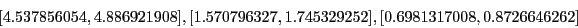The system has a solution which is approximatively: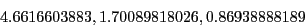This problem is extremely ill conditioned as for the TestDomain the functions intervals are: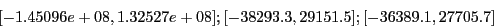The name of the test program is Test_Solve_General_Gradient. With espsilonf=0 and epsilon=0.001 and if we stop at the first solution we find with the maximum equation ordering: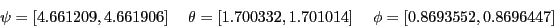with 73 boxes using the direct storage mode (with the reverse storage mode only 37 boxes are needed). With the maximum middle-point equation ordering we find the same intervals with 67 boxes in the direct storage mode (41 for the reverse storage mode).

The importance of normalizing the functions has been mentioned in section 2.3.5.4. But in this example the use of the Jacobian matrix enable to drastically reduce the computation time. If we use epsilonf=0.1 and epsilon=0 and if we stop at the first solution we find an exact solution using 73 boxes: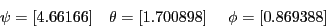in a time which is about 1/100 of the time necessary when we don't use the Jacobian.

In the single bisection smear mode (i.e. only one variable is bisected in the process) the same root is obtained in 21080ms (about 50% less time than when using the full bisection mode) with only 40 boxes in the direct storage mode.

Note that for epsilonf=0.1 and epsilon=0 we find the only root with 73 boxes in 39760ms (41 boxes and 24650ms in the single bisection smear mode).

Note that we may improve the efficiency of the procedure by using simplification procedures such as the 2B (section 2.17) and the 3B method. An interesting point in this example is that the bisection mode 1 (bisecting along the variable whose interval has the largest diameter) is more effective than using the bisection mode 2 (using the smear function) with 53 boxes against 108 for the mode 2 for epsilonf=1e-6 and epsilon=1e-6. This can easily been explained by the complexity of the Jacobian matrix elements that leads to a large overestimation of their values when using interval: in that case the smear function is not very efficient to determine which variable has the most influence on the equations.Next: General comments Up: Examples Previous: Example 3   Contents
Jean-Pierre Merlet 2012-12-20